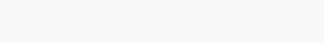# Insert Delete GetRandom O(1) | Solution Explained

LeetCode 380. Insert Delete GetRandom O(1) is an implementation based question, requiring us to know the time complexities of simple data structures like lists and sets. Problem Link.

## Insert Delete GetRandom: Inefficient Version

• my first instinct was to use sets.
• it works great for
• insert
• remove
• but it fails in the case of `getRandom`, where we’ll have a type errortype error for random.choice on sets for Insert Delete GetRandom

The only way to get around it, is to use `random.choice(list(set))`, which is going to convert the entire list into a set. But, this takes O(N) time.

```class RandomizedSet:
def __init__(self):
self.s = set()

def insert(self, val: int) -> bool:
if val in self.s:
return False
return True

def remove(self, val: int) -> bool:
if val in self.s:
self.s.remove(val)
return True
return False

def getRandom(self) -> int:
return random.choice(list(self.s))
```

## Insert Delete GetRandom: Optimized

• we need a list, `data`, that stores all the items in a list
• since search and removal is inefficient in list, we workaround by using another dictionary for O(1) retrieval of information – is the `val` in the list or not?
```class RandomizedSet:

def __init__(self):
self.data = []
self.d = {}

def insert(self, val: int) -> bool: #O(1)
if val in self.d: return False

self.d[val] = len(self.data)
self.data.append(val)
return True

def remove(self, val: int) -> bool:
if val not in self.d: return False

index = self.d[val]
last = self.data[-1]
curr = self.data[index]

self.data[index] = last
self.data[-1] = curr
self.data.pop()

self.d[last] = index
del self.d[curr]

return True

def getRandom(self) -> int: # O(1)
return random.choice(self.data)
```